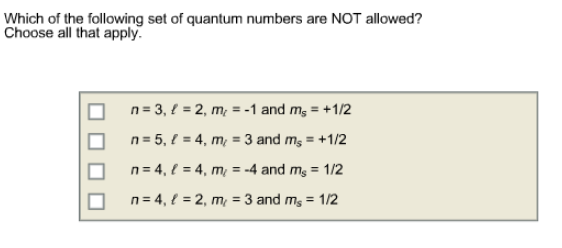# Problem: Which of the following set of quantum numbers are NOT allowed? Choose all that apply.a. n = 3, l = 2, m_l = -1 and m_s = +1/2 b. n = 5, l = 4, m_l = 3 and m_s = + 1/2 c. n = 4, l = 4, m_l = -4 and m_s = 1/2 d. n = 4, l = 2, m_l = 3 and m_s = 1/2

###### FREE Expert Solution

The things you have to remember in answering this type of question is that l =n-1 or less, ml = +/- l or less and ms is just +/- 1/2.

From that,
c. n = 4, l = 4, m_l = -4 and m_s = 1/2
violates the rule that l should be at most 1 less than n. For n=4, maximum l =3.

93% (91 ratings)###### Problem Details

Which of the following set of quantum numbers are NOT allowed? Choose all that apply.

a. n = 3, l = 2, m_l = -1 and m_s = +1/2

b. n = 5, l = 4, m_l = 3 and m_s = + 1/2

c. n = 4, l = 4, m_l = -4 and m_s = 1/2

d. n = 4, l = 2, m_l = 3 and m_s = 1/2